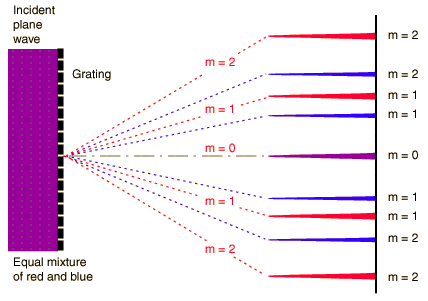# Focused diffraction and Fourier transform

#### Choisai

After searching on the web and reading a bit, I found that lenses can perform Fourier transform. All you need to do is put a transparant object in front of it, like a transparant sheet with black stripes on it and a screen behind the lens(so basically a 4f setup). The lens will then perform a Fourier transform and voila, you will see three dots on the screen after the lens.

The center is the average brightness and the other two dots next to it stand for the spatial frequency of the object (two dots meaning ofcourse that there is one spatial frequency).

How can a lens do this? It certainly doesn't take a sheet of paper and start doing calculations to transform the light into the bright spots.
I found an explanation of this in a video of Youtube channel 'Applied Sciences'. In the video 'intro to Fourier optics' he states that the Fourier transform of the lens is actually the focussing of diffraction.

The transparant sheet acts like a grating and causes diffraction. The lens simply focusses this diffraction, which results in the Fourier transform. Meaning that diffraction and Fourier transforms are simply two sides of the same coin. One is a mathematical description, while the other is a 'physical' explanation (physics = applied maths but you know what I mean here).

But my question is this:
If the Fourier transformation as carried out by a lens is simply the focussing of the diffraction, then why don't we see more dots? Diffraction has many bright spots: the zeroth order (the one that is unchanged as if there was no sheet), the first order, but also the second order, the third, the fourth etcetera. Yet only the first and zeroth order of diffraction shows up.

So why don't we see those higher orders?

#### UltrafastPED

Gold Member
The mathematics study of a lens and a wavefront shows that the lens performs a "spatial Fourier transform" on the wavefront.

See "Fourier Optics" for details: http://www.optics.rochester.edu/workgroups/george/FO_BOOK.pdf

Most optics text books give at least some treatment to this subject.

Unsurprisingly, this also applies to electron optics - a transmission electron microscope can show you the diffraction pattern or the image by simply changing the focal plane. For electron optics the typical lens is magnetic, so you only need to change a current!

See http://www.rodenburg.org/theory/y1300.html

So you can see that an analysis of diffraction is crucial to this analysis.

#### Andy Resnick

<snip>

But my question is this:
If the Fourier transformation as carried out by a lens is simply the focussing of the diffraction, then why don't we see more dots? Diffraction has many bright spots: the zeroth order (the one that is unchanged as if there was no sheet), the first order, but also the second order, the third, the fourth etcetera. Yet only the first and zeroth order of diffraction shows up.

So why don't we see those higher orders?
Recall that your setup images the far-field diffraction pattern of the object at the back focal plane of the lens. If your object is a Ronchi ruling (or an amplitude 'square wave'), there are many spatial frequencies present and then you should see many 'dots'. If the amplitude transmittance of your object is a pure sine, then there's only one spatial frequency. If your object introduces a sine-wave modulation of phase rather than amplitude (a phase-only object), the diffraction pattern will again have many features.

#### Choisai

Recall that your setup images the far-field diffraction pattern of the object at the back focal plane of the lens. If your object is a Ronchi ruling (or an amplitude 'square wave'), there are many spatial frequencies present and then you should see many 'dots'. If the amplitude transmittance of your object is a pure sine, then there's only one spatial frequency.
The grating here has a single spatial frequency. The picture shows the first order, but a grating also produces the second and third order. That is why a grating also produces this cool effect:Here the light isn't monochromatic, but the grating still produces higher orders. Why aren't these shown in 4f images? Is it because they don't exist? Or is it that they do exist but they are not useful and therefore left out of such drawings?

EDIT: I just came across 'sinus gratings' and 'bar gratings'. Do the bar gratings have multiple spatial frequencies because of their binary nature (because they are effectively square waves)?

If so, that would explain a lot

Last edited by a moderator:

#### dauto

EDIT: I just came across 'sinus gratings' and 'bar gratings'. Do the bar gratings have multiple spatial frequencies because of their binary nature (because they are effectively square waves)?
Yes, the Fourier transform of a square wave has higher harmonics while the transform of a sine wave doesn't.

### The Physics Forums Way

We Value Quality
• Topics based on mainstream science
• Proper English grammar and spelling
We Value Civility
• Positive and compassionate attitudes
• Patience while debating
We Value Productivity
• Disciplined to remain on-topic
• Recognition of own weaknesses
• Solo and co-op problem solving